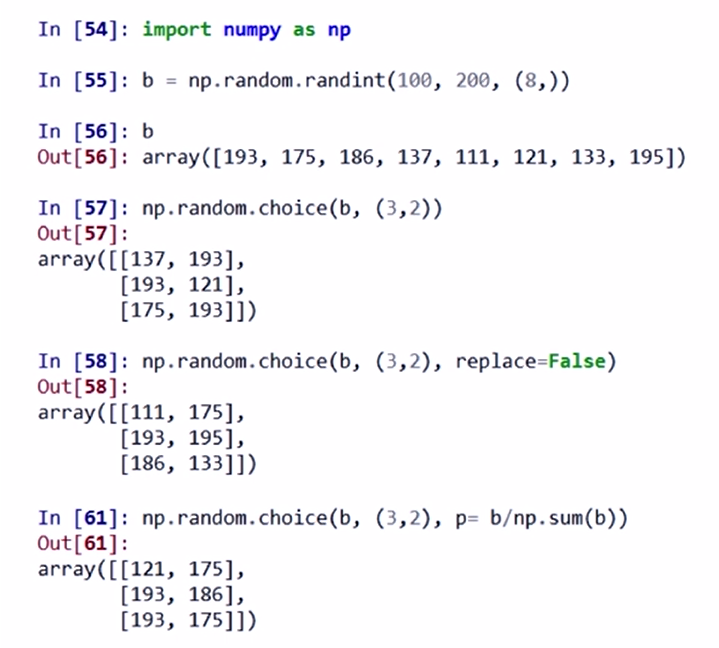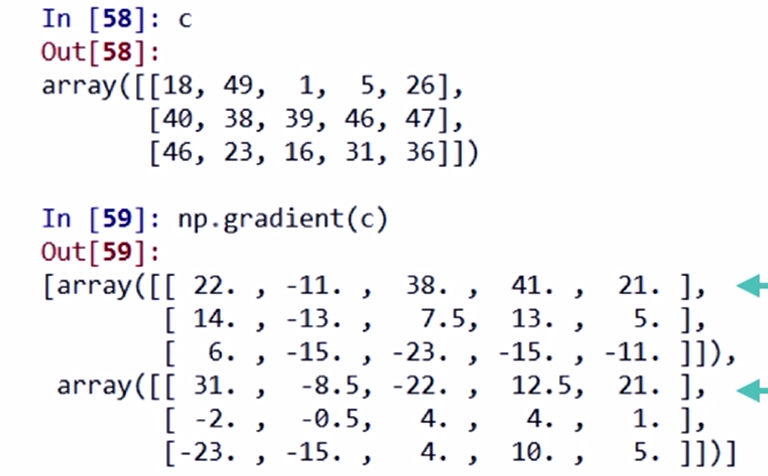# numpy大全（一直补充）

import numpy as np

.ndim ：维度

.shape ：各维度的尺度 ，例如简单的二维（5×5）显示为[5， 5]

.size ：元素的个数 10
.dtype ：元素的类型 dtype(‘int32’)
.itemsize ：每个元素的大小，以字节为单位 ，每个元素占4个字节
ndarray：数组的创建
np.arange(1, 11).reshape([5, 2]) #生成1~10的等差数列并重新排列为5×2的数组
np.ones(shape): 生成全1  np.ones([5, 5]) #生成为1的5×5数
np.zeros((shape)， ddtype = np.int32) ： 生成int32型的全0 ,np.zeros([4, 4]) #生成为0的4×4数组
np.full(shape, val): 生成全为val
np.eye(n) : 生成单位矩阵,n表示nxn

np.ones_like(a) : 按数组a的形状生成全1的数组
np.zeros_like(a): 同理
np.full_like (a, val) : 同理

np.linspace（1,10,4）： 根据起止数据等间距地生成数组
np.linspace（1,10,4, endpoint = False）：endpoint 表示10是否作为生成的元素
np.concatenate():numpy提供了numpy.concatenate((a1,a2,…), axis=0)函数。能够一次完成多个数组的拼接。其中a1,a2,…是数组类型的参数

• 数组的维度变换

.reshape(shape) : 不改变当前数组，依shape生成       shape=[n,m]
.resize(shape) : 改变当前数组，依shape生成
.swapaxes(ax1, ax2) : 将两个维度调换
.flatten() : 对数组进行降维，返回折叠后的一位数组

• 数组的类型变换

• 一维数组切片

a = np.array ([9, 8, 7, 6, 5, ])
a[1:4:2] –> array([8, 6]) ： a[起始编号：终止编号（不含）： 步长]

• 多维数组索引

a = np.arange(24).reshape((2, 3, 4))
a[1, 2, 3] 表示 3个维度上的编号， 各个维度的编号用逗号分隔

• 多维数组切片

a [：，：，：：2 ] 缺省时，表示从第0个元素开始，到最后一个元素

np.abs(a) np.fabs(a) : 取各元素的绝对值
np.sqrt(a) : 计算各元素的平方根
np.square(a): 计算各元素的平方
np.log(a) np.log10(a) np.log2(a) : 计算各元素的自然对数、10、2为底的对数
np.ceil(a) np.floor(a) : 计算各元素的ceiling 值， floor值（ceiling向上取整，floor向下取整）
np.rint(a) : 各元素 四舍五入
np.modf(a) : 将数组各元素的小数和整数部分以两个独立数组形式返回
np.exp(a) : 计算各元素的指数值
np.sign(a) : 计算各元素的符号值 1（+），0，-1（-）
np.maximum(a, b) np.fmax() : 比较（或者计算）元素级的最大值
np.minimum(a, b) np.fmin() : 取最小值
np.mod(a, b) : 元素级的模运算

np.copysign(a, b) : 将b中各元素的符号赋值给数组a的对应元素

CSV (Comma-Separated Value,逗号分隔值) 只能存储一维和二维数组

1.np.savetxt(frame, array, fmt=’% .18e’, delimiter = None):

frame是文件路径、字符串等，可以是.gz .bz2的压缩文件； array 表示存入的数组； fmt 表示元素的格式 eg： %d % .2f % .18e ; delimiter： 分割字符串，默认是空格

eg： np.savetxt(‘a.csv’, a, fmt=%d, delimiter = ‘,’ )

2.np.loadtxt(frame, dtype=np.float, delimiter = None, unpack = False) :

frame是文件路径、字符串等，可以是.gz .bz2的压缩文件； dtype：数据类型，读取的数据以此类型存储； delimiter: 分割字符串，默认是空格; unpack: 如果为True， 读入属性将分别写入不同变量。

3.多维数据的存取

（1）a.tofile(frame, sep=’’, format=’%s’ ) :

frame: 文件、字符串； sep: 数据分割字符串，如果是空串，写入文件为二进制 ； format:： 写入数据的格式  eg: a = np.arange(100).reshape(5, 10, 2)      a.tofile(“b.dat”, sep=”,”, format=’%d’)

（2）np.fromfile(frame, dtype = float, count=-1, sep=’’)： frame： 文件、字符串 ； dtype： 读取的数据以此类型存储； count：读入元素个数， -1表示读入整个文件； sep: 数据分割字符串，如果是空串，写入文件为二进制

PS: a.tofile() 和np.fromfile（）要配合使用，要知道数据的类型和维度。

np.save(frame, array) : frame: 文件名，以.npy为扩展名，压缩扩展名为.npz ； array为数组变量

numpy 的random子库

rand(d0, d1, …,dn) : 各元素是[0, 1）的浮点数，服从均匀分布
randn(d0, d1, …,dn)：标准正态分布
randint(low， high,（ shape）): 依shape创建随机整数或整数数组，范围是[ low, high）
seed(s) ： 随机数种子

shuffle(a) : 根据数组a的第一轴进行随机排列，改变数组a
permutation(a) : 根据数组a的第一轴进行随机排列， 但是不改变原数组，将生成新数组
choice(a[, size, replace, p]) : 从一维数组a中以概率p抽取元素， 形成size形状新数组，replace表示是否可以重用元素，默认为False。
eg：replace = False时，选取过的元素将不会再选取

uniform(low, high, size) : 产生均匀分布的数组，起始值为low，high为结束值，size为形状
normal(loc, scale, size) : 产生正态分布的数组， loc为均值，scale为标准差，size为形状
poisson(lam, size) : 产生泊松分布的数组， lam随机事件发生概率，size为形状

eg: a = np.random.uniform(0, 10, (3, 4)) a = np.random.normal(10, 5, (3, 4))

sum(a, axis = None) : 依给定轴axis计算数组a相关元素之和，axis为整数或者元组
mean(a, axis = None) : 同理，计算平均值
average(a, axis =None, weights=None) : 依给定轴axis计算数组a相关元素的加权平均值
std（a, axis = None） ：同理，计算标准差
var（a, axis = None）: 计算方差
eg： np.mean(a, axis =1) ： 对数组a的第二维度的数据进行求平均
a = np.arange(15).reshape(3, 5)
np.average(a, axis =0, weights =[10, 5, 1]) : 对a第一各维度加权求平均，weights中为权重，注意要和a的第一维匹配

min(a) max(a) : 计算数组a的最小值和最大值
argmin(a) argmax(a) : 计算数组a的最小、最大值的下标（注：是一维的下标）
unravel_index(index, shape) : 根据shape将一维下标index转成多维下标
ptp(a) : 计算数组a最大值和最小值的差
median(a) : 计算数组a中元素的中位数（中值）
eg：a = [[15, 14, 13],
[12, 11, 10] ]
np.argmax(a) –> 0

np.unravel_index( np.argmax(a), a.shape) –> (0,0)PIL， python image library 库
from PIL import Image
Image是PIL库中代表一个图像的类（对象）

im = np.array(Image.open(“.jpg”))

im = Image.fromarray(b.astype(‘uint8’)) # 生成
im.save(“路径.jpg”) # 保存

im = np.array(Image.open(“.jpg”).convert(‘L’)) # convert(‘L’)表示转为灰度图